﻿

### Questioni di suriettività di un morfismo canonico tra due complessi approssimati

#### Abstract

In this paper, we introduce the concept of i-couple for two ideals,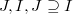$J,I, J\supseteq I$, in a local noetherian ring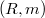$(R,m)$.This concept is expressed in terms of the structure of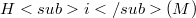$Hi(M)$ (where M is an approximation complex for the double Koszul complex L associated to the system of generators of J), and it generalizes the idea of (d,i)-sequence introduce in [M-R].We study the relationship between the following properties: 1)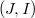$(J,I)$ is an i- couple of ideals in R; 2)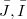$\bar{J},\bar{I}$ is an i- couple of ideals in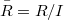$\bar{R}=R/I$, more generally, in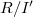$R/I'$,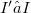$I'⊆ I$.So we get some sufficient conditions for the "ascendent" and "descendent" properties of the i –couple.In particular, we study the surjectivity of the natural morphism(Error rendering LaTeX formula), since the surjectivity of$\bar{φ}i$ is a sufficient condition for the "descendent" property of the i - ouple from R to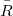$\bar R$.

DOI Code: 10.1285/i15900932v6n1p61

Full Text: PDF